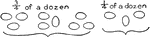### Dozen in Fourths

Visual of eggs showing 3/4 of a dozen and 1/4 of a dozen. 3x4=12, a dozen.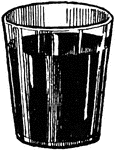### Three Quarters Full Glass

An illustration of a glass that is three quarters full.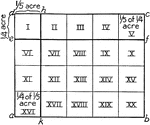### Mixed Numbers

"Here we see 1/2 of 3/4 lb. of candy= 3/8 lb. candy. Use inch, cubes and pennies to show such relations.…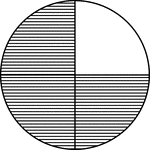### Fraction Pie Divided into Quarters

A circle divided into quarters with three quarters shaded.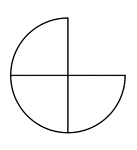### Three Quarters of a Fraction Pie

Three quarters of a circle.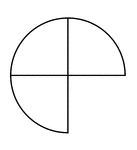### Three Quarters of a Fraction Pie

Three quarters of a circle.### Three Quarters of a Fraction Pie

Three quarters of a circle.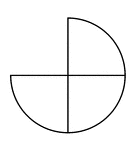### Three Quarters of a Fraction Pie

Three quarters of a circle.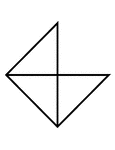### Fractions of 4-sided Polygon

3/4 of a 4 sided polygon